# Highlights

• Novel multi-view autoencoder architecture (Shape MAE) which learns latent representation of cardiac shapes from multiple standard views
• Multi-view UNet that can incorporate anatomical shape priors to guide the segmentation of short-axis images
• More robust than 2D or 3D segmentation baselines when using less training data (10%)

# Introduction

A number of methods already improve the robustness of cardiac segmentations, but they have some drawbacks:

• Heavy computational cost (ensemble model) or
• Requirement of fully annotated 3D high-resolution images, without inter-slice motion artifacts (often not feasible for patients with cardiovascular diseases)

# Methods

Given a source view $$X_i$$, the network learns the low-dimensional representation $$z_i$$ of $$X_i$$ that best reconstructs all the $$j$$ target views segmentations $$Y_j$$.

The authors use four source views $$X_i$$ ($$i = 1, \dots, 4$$) which are three long axis (LA) images - the two-chamber view (LA1), three-chamber view (LA2), the four-chamber view (LA3) - and one mid-ventricular slice (Mid-V) from the short axis (SA) view.

The target segmentations views $$Y_j$$ ($$j = 1, \dots, 6$$) correspond to the four previous views plus two SA slices: the apical one and the basal one.

The Shape MAE architecture is detailed in Figure 1.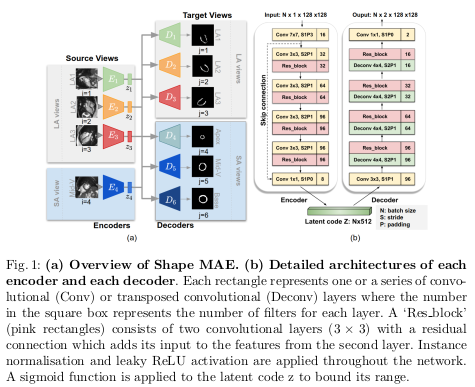The global loss for training the Shape MAE is shown in equation 1.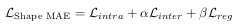where:

• $$L_{intra}$$ denotes the segmentation loss when the source view $$X_i$$ and the target view $$Y_j$$
• $$L_{inter}$$ denotes the loss when two views differ in the number of filters at each level by four times to account for the fact that cardiac segmentation is simpler than the lesion segmentation
• $$\alpha$$ and $$\beta$$ are not both set to 1! $$\alpha$$ was empirically set to 0.5 and $$\beta$$ to 0.001.

As for the proposed multi-view U-Net (MV U-Net), it is based on the original U-Net. The number of filters was reduced at each level by four times to account for the fact that cardiac segmentation is simpler than the original U-Net task (lesion segmentation with multiple candidates). A module called ‘Fuse Block’ is also introduced in the bottleneck of the U-Net to merge the latent spaces with the feature maps from the U-Net. The detailed architecture is shown in Figure 2.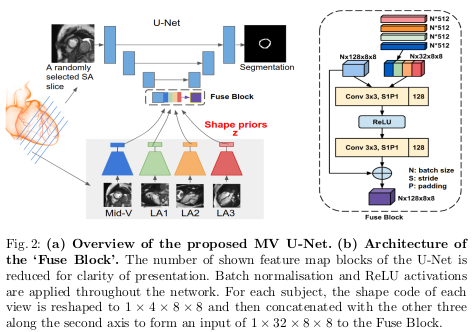## Data

Experiments were performed on a dataset acquired from 734 subjects (from the UK Biobank). The training set consists of 570 patients. For each subject, a stack of 2D SA slices and three orthogonal 2D LA images are available. All the LV myocardium were annotated on the SA images as well as the LA images at the end-diastolic (ED) frame using an automated method followed by manual quality control.

# Results

The quantitative results of the proposed architecture are shown in Table 1, while examples of increased robustness on edge cases are shown in Figure 3.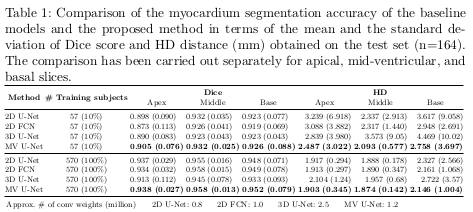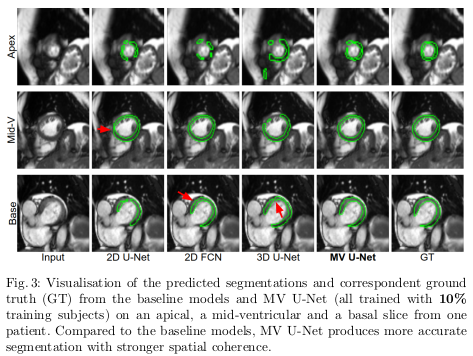# Conclusions

The proposed method achieves high segmentation performance with limited training data, but no significant improvements are shown when compared to baselines trained on the whole dataset (even if the authors claim the 0.01 increase in dice is significative).

# Remarks

• The authors claim the “approach does not require a dedicated acquisition protocol, since LA images are routinely acquired in most CMR imaging schemes.”